# Math 113 Problem Set 7

Due October 31, 2003

1. In class we found that the function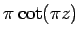has a pole at every integer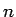with residue 1. One natural approach to constructing such a function is to consider a sum over the integersof a function with a pole at: consider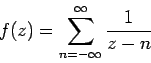(1)

1. Show that this approach does not work, by showing that the sum above diverges for all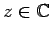.

(Recall that a sum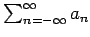converges if and only if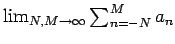exists, which in turn is true if and only if there is a value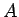so that for every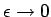, there are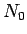and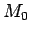so that, for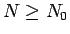and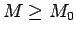,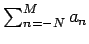is within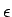of the limiting value.)

We can be a little more clever, by taking both endpoints of the sum simultaneously to infinity: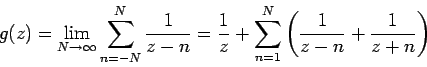(2)

1. Show that the sum in (2) converges, and that the result is an analytic function with a pole at every integer with residue 1 which satisfies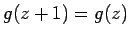.
2. Evaluate the sum to show that, in fact,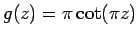.
In summary, althoughis not the only function with a pole of residue 1 at each integer, it is in some sense the most natural one.
2. Show that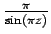has a pole at every integer, with a residue atof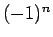.
3. Bak and Newman, Chapt. 11, p. 147, problem 6.
4. Bak and Newman, Chapt. 11, p. 147, problem 5.
5. Bak and Newman, Chapt. 11, p. 147, problem 1(d).
6. Needham, p. 446, problem 3.
7. Needham, p. 446, problem 1.
8. (Optional) Needham, p. 447, problem 4.

Dylan Thurston 2003-10-27